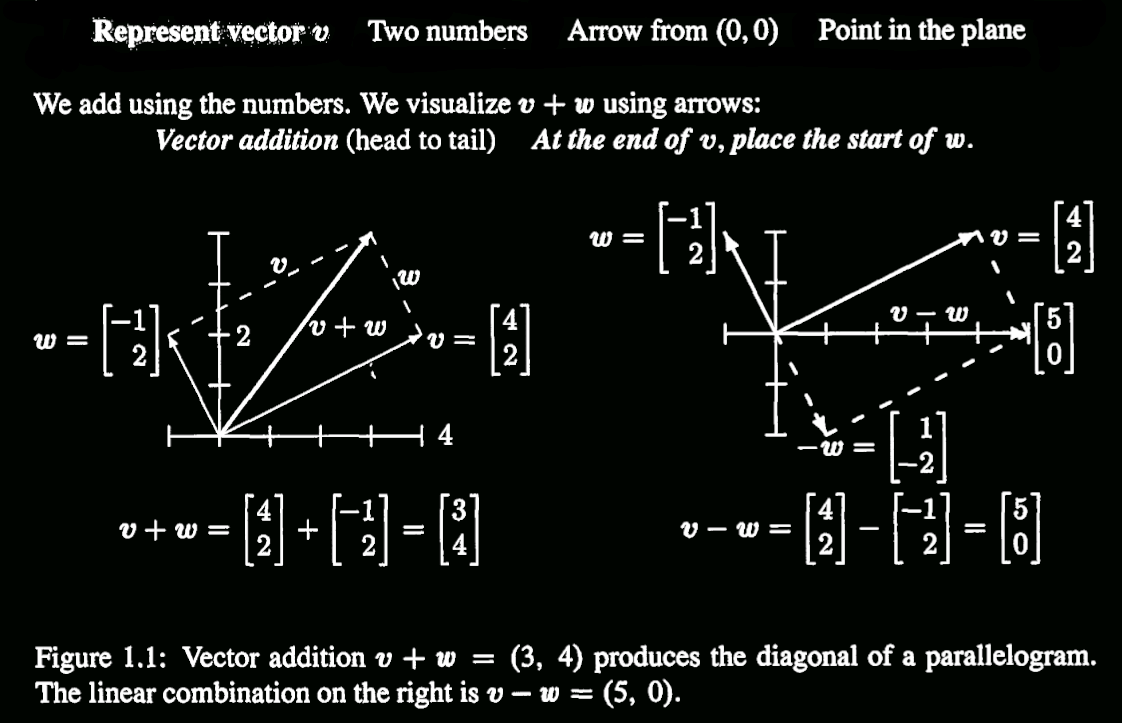Abstract: 线性组合详细说明
Keywords: Linear Combinations

# 线性组合

## 列向量

like this one：
$$\begin{bmatrix} 4\\5 \end{bmatrix}$$

## 向量加法和乘法计算

$$\textbf{v}=\begin{bmatrix} v_1\\v_2 \end{bmatrix}\\ \textbf{w}=\begin{bmatrix} w_1\\w_2 \end{bmatrix}\\$$
$$\textbf{v}+\textbf{w}=\begin{bmatrix} v_1+w_1\\v_2+w_2 \end{bmatrix}\\$$

VECTOR MULTIPLICATION:
$$2\textbf{v}=\begin{bmatrix} 2v_1\\2v_2 \end{bmatrix}\\ -\textbf{v}=\begin{bmatrix} -v_1\newline -v_2 \end{bmatrix}\\$$
（写公式真累！！）

## 线性组合

$$c\textbf{v}+d\textbf{w}\\$$

the sum of $c\textbf{v}$ and $d\textbf{w}$ is a linear combination of $\textbf{v}$ and $\textbf{w}$

## 向量的表示## Important Questions

Suppose
$\textbf{u}$ $\textbf{v}$ $\textbf{w}$ are three-dimensional non-zero:

$c\textbf{u}$ fill a Linear

$c\textbf{u}+d\textbf{v}$ fill a plane

$c\textbf{u}+d\textbf{v}+e\textbf{w}$ fill a space(3d)

0%